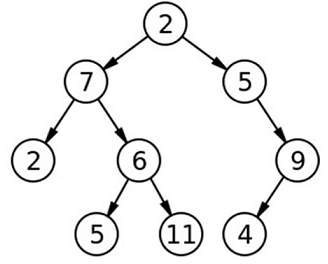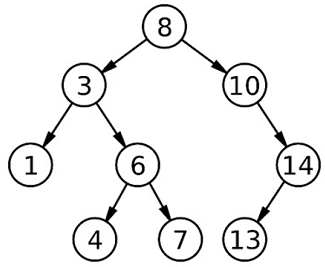# Tree Data structure

Data Structure | Tree: In this tutorial, we are going to learn about the tree data structure, tree structure, tree definition, different types of trees, applications of trees, tree tutorials.
Submitted by Radib Kar, on July 24, 2020

Tree is one of the most popular non-linear data structures often used to implement business logic and to store data. There have been several advantages of tree data structure and thus it has huge applications in computer science fields. Most of the product based companies always check where the interviewee has a strong knowledge of tree or not. As per theoretical knowledge is concerned, one need to be strong with solving standard tree problems too. That's why here we have listed all tree tutorials as wee as tree coding problems which have been featured in many interview rounds. We have listed all standard tree problems which stand on the basic ground of handing tree problems using tree traversals, conversions and of course using symmetry in a tree.

As we said being a non-linear data structure, Tree is very useful in case of storing data and retrieving data in less time complexity. Average tree ADT operations like insertions, deletion, searching takes time of O(h) where h is the height of the tree. This reduces the time complexity heavily since the height of the tree often comes in logarithmic range. For example, if the tree is a binary tree and balanced tree, then normally the height becomes O(log2n) where n is the number of nodes.

## Tree Structure

Normally, in general, a tree consists of its root and its children. Tree follows symmetric nature and that means the children are themselves trees known as subtrees and follow the same definition like a tree. Each node stores some data and pointer/link to its children.

```Structure TreeNode{
T data;
List<TreeNode> children;
}
```

Below is the example of a tree,In general, a tree can have any number of children. There may be some nodes which have no children(otherwise it would become an infinite tree), known as a leaf node.

## Tree Definitions

1. Root:
Root is the node from where every tree starts which is at height 0. Like for the above example node with value 2 is the root. A root can have any number of children from 0 to n. A tree is defined by its root. From the root, we can traverse the entire tree.
2. Children:
Every tree node has some children who are themselves tree(subtree). Like in the above example tree root 2 has two children. One is a subtree rooted with 7 and another is a subtree rooted with 5.
3. Subtree:
Subtree means children in the tree. Each child is either a subtree or a leaf node.
4. Leaf node:
A node with no child is known as a leaf node. All its child are marked as NULL.

## Different Types of Trees in Data Structure

1. Binary tree:
Binary tree is the most used tree where a node can have at most 2 children. Binary tree has again three variations namely complete binary tree, full binary tree & perfect binary tree.
In binary tree, children are known as left child and right child. Either can be subtree or Leaf node or NULL.
2. Ternary tree:
Ternary tree is another variation of tree data structure where a node can have at most 3 children.
3. N-array tree:
N-array tree is the generic tree having any number of child. On the other hands it's an undirected graph without any cycle
4. Binary search tree:
Binary search tree is a variation of binary tree where the root and its child has some ordering for storing data. For binary search tree, the rule is that all the values in the left child(can be a subtree as well) will be less than the root value and all the values in the right child(can be a subtree as well) will be greater than the root value. And this will satisfy for recursively for any subtree. Below is an example of BST.5. Skew tree:
While building up a BST, we may find that the tree becomes skewed and then it becomes like a linked list almost as height becomes O(n) instead of O(logn). This type of BSTs are known as skew tree and this is not at all entertained( Because we lose the advantage of tree data structure here as it becomes a linear data structure.
6. Self-balancing tree:
To avoid the problem of the skew tree, self-balancing tree is introduced where the difference b/w right and left subtree will never become more than 1. Some example of such trees is AVL tree, Red-black tree, splay tree etc.

There are also some advance tree data structures often helps in solving problems optimally like segment tree, Trie, Binary index tree, Suffix tree., ScapeGoat Tree, Treap etc which we will provide tutorials on.

## Application of Tree

1. ADT operations like insertion, deletion, searching are faster in trees and thus it’s often used to store data.
2. File system uses tree hierarchy
3. B-tree is used in DBMS to implement indexing
4. Compiler forms a syntax tree
5. Heap is also a tree which is used for priority queue
6. Trie is used for faster word dictionary implementation
7. HTML, XML are also stored as tree. (Render tree)

## Tree Tutorials

Basics:

Tree Traversals:

Tree Construction and Conversion:

Binary Tree Summations:

Binary Tree | Checking & Printing

Tree coding problems (programs on tree data structure)